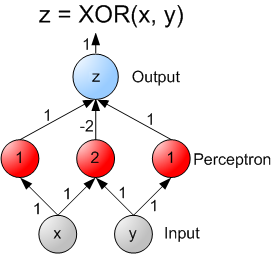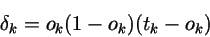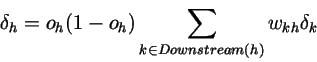2 views

I'm trying to understand the backpropagation algorithm with an XOR neural network as an example. In this case, there are 2 input neurons + 1 bias, 2 neurons in the hidden layer + 1 bias, and 1 output neuron.

A B A XOR B 1 1 -1 1 -1 1 -1 1 1 -1 -1 -1(source: wikimedia.org)

I'm using stochastic backpropagation.

After reading a bit more I have found out that the error of the output unit is propagated to the hidden layers... initially this was confusing, because when you get to the input layer of the neural network, then each neuron gets an error adjustment from both of the neurons in the hidden layer. In particular, the way the error is distributed is difficult to grasp at first.

Step 1 calculate the output for each instance of input.

Step 2 calculate the error between the output neuron(s) (in our case there is only one) and the target value(s):Step 3 we use the error from Step 2 to calculate the error for each hidden unit h:The 'weight kh' is the weight between the hidden unit h and the output unit k, well this is confusing because the input unit does not have a direct weight associated with the output unit. After staring at the formula for a few hours I started to think about what the summation means, and I'm starting to come to the conclusion that each input neuron's weight that connects to the hidden layer neurons is multiplied by the output error and summed up. This is a logical conclusion, but the formula seems a little confusing since it clearly says the 'weight kh' (between the output layer k and hidden layer h).

Am I understanding everything correctly here? Can anybody confirm this?

What's O(h) of the input layer? My understanding is that each input node has two outputs: one that goes into the first node of the hidden layer and one that goes into the second node hidden layer. Which of the two outputs should be plugged into the O(h)*(1 - O(h)) part of the formula?by (33.1k points)

In the Backpropagation algorithm, we search for derivatives of the error function w.r.t. to a unit or weight. To understand this algorithm, you have to understand the chain rule in derivatives. You need an understanding of calculus to understand derivatives.

∂E/∂W can be composed of ∂E/∂o ∂o/∂W using the chain rule. ∂o/∂W can easily be calculated. The derivative of the activation/output of a unit w.r.t. the weights. ∂E/∂o is known as deltas.

We use them on outputs so that we can calculate the error. If the error is above the threshold, then the output

The output of a unit is the sum of all incoming units weighted by their weights and the application of a transfer function afterward.

So

o_k = f(sum(w_kj * o_j, for all j)).

You can derive o_k with respect to o_j. Since delta_j = ∂E/∂o_j = ∂E/∂o_k ∂o_k/∂o_j = delta_k ∂o_k/o_j. Then delta_k, we can calculate delta_j!

o_k = f(sum(w_kj * o_j, for all j)) => ∂o_k/∂o_j = f'(sum(w_kj * o_j, for all j)) * w_kj = f'(z_k) * w_kj.

The sigmoidal transfer function (STF), this becomes

z_k(1 - z_k) * w_kj.

The author says

o_k(1 - o_k) * w_kj!)